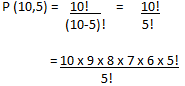# Engineering Maths - Permutation And Combinations

>>>>>>>>Permutation And Combinations

• A

20• B

35• C

40• D

30• A

20120• B

30120• C

30240• D

40840• Option : C
• Explanation : Total letters in the word "LOGARITHMS" is 10,i.e. n=10 and we have to form five-letter words
r = 5 Required number of words,= 10 x 9 x 8 x 7 x 6

= 30240

• A

3• B

4• C

6• D

9• Option : B
• Explanation : Since npr is product of consecutive positive integers.

npr = 3024 = 9 x 8 x 7 x 6

Hence,        r = 4

• A

20• B

30• C

60• D

120• Option : C
• Explanation : First three jobs can be filled by 5 members in 5P3 ways.

Number of ways of filling the first 3 jobs

5P= 5 x 4 x 3 = 60 ways.

• A

120• B

240• C

1200• D

14400